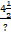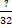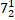# Quiz Discussion

One-fourth of a herd of camels was seen in the forest. Twice the square root of the herd had gone to mountains and the remaining 15 camels were seen on the bank of a river. Find the total number of camels ?

Course Name: Quantitative Aptitude

• 1] 32
• 2] 34
• 3] 35
• 4] 36
##### Solution
No Solution Present Yet

#### Top 5 Similar Quiz - Based On AI&ML

Quiz Recommendation System API Link - https://fresherbell-quiz-api.herokuapp.com/fresherbell_quiz_api

# Quiz
1
Discuss

How many perfect squares lie between 120 and 300 ?

• 1] 5
• 2] 6
• 3] 7
• 4] 8
##### Solution
2
Discuss

$${\left[ {{{\left( {\sqrt {81} } \right)}^2}} \right]^2} = {\left( ? \right)^2}$$

• 1] 8
• 2] 9
• 3] 4096
• 4] 6561
• 5] None of these
##### Solution
3
Discuss

$$\sqrt {1.5625} = ?$$

• 1] 1.05
• 2] 1.25
• 3] 1.45
• 4] 1.55
##### Solution
4
Discuss

Which number can replace both the question marks in the equation=?

• 1]

12

• 2]

7

• 3]• 4]

None of these

##### Solution
5
Discuss

The square root of $$\left( {{{272}^2} - {{128}^2}} \right)$$  is = ?

• 1] 144
• 2] 200
• 3] 240
• 4] 256
##### Solution
6
Discuss

If √x+x/y  = x√x/y  where x and y are positive real numbers, then y is equal to ?

• 1]

x + 1

• 2]

x - 1

• 3]

x2 + 1

• 4]

x2 - 1

##### Solution
7
Discuss

R is a positive number. It is multiplied by 8 and then squared. The square is now divided by 4 and the square root is taken. The result of the square root is Q. What is the value of Q ?

• 1] 3R
• 2] 4R
• 3] 7R
• 4] 9R
##### Solution
8
Discuss

$$\sqrt {\frac{{16}}{{25}}} \times \sqrt {\frac{?}{{25}}} \times \frac{{16}}{{25}} = \frac{{256}}{{625}}$$

• 1] 5
• 2] 8
• 3] 16
• 4] None of these
##### Solution
9
Discuss

$$\sqrt ? \times \sqrt {484} = 1034$$

• 1] 2025
• 2] 2209
• 3] 2304
• 4] 2401
• 5] None of these
##### Solution
10
Discuss

The square root of 123454321 is = ?

• 1] 111111
• 2] 12341
• 3] 11111
• 4] 11211
# Quiz